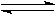Titration of a strong acid by a strong base

The applet below draws the graph of pH against volume of titrant added for the titration of strong acid by a strong base. Specifically:

 a solution of 0.1 mol/L NaOH (aq) is the titrant added from a burette 25 cm3 of a solution of 0.1 mol/L HCl (aq) is the analyte in a flask below the burette titrant is added beyond the equivalence point when 25 cm3 of titrant has been added. turn on the data table feature to see the pH value of the equivalence point.

Press the run button to draw the pH curve for the strong acid/strong base titration.

Message: Java Applet not loaded !

If you were using a Java-enabled browser ,you would see pH curve plotter program here instead of this paragraph.

Check your browser options list to enable Java programs to run in this browser.

Note that for this titration:

 the equivalence point is at exactly pH 7.0

The shape of the graph is explained as follows:

Before the equivalence point:

Added OH-(aq) present in the NaOH(aq) titratant is neutralised by the H3O+(aq) in the analyte solution to produce water:

OH-(aq) + H3O+(aq) --> 2H2O(l)

Na+ (from the NaOH solution) and Cl- (from the HCl solution) are spectator ions in the process. The overall result is that the added base is neutralised, acid is consumed and a solution of the salt sodium chloride, NaCl(aq), is produced.

The resulting pH is simply given by [H3O+], the concentration of hydronium ions remaining:

pH = -log10[H+]

Where [H+] is equivalent to [H3O+].

At the equivalence point:

All the acid has just been neutralised by the base to give a solution of sodium chloride in water only. The only source of hydronium ions is through the dissociation of water:

2H2O(l)H3O+(aq) + OH-(aq)

given that:

[H3O+] x [OH-] = 1 x10-14 mol2/L2

and that:
[H3O+] = [OH-]

= 1 x 10-7 mol/L

using:
pH = -log10[H+]

= 7

After the equivalence point:

The pH of the solution is determined by the equilibrium for the dissociation of water with the concentration of H3O+(aq) being progressively decreased as the increasing concentration of OH-(aq) shifts the equilibrium to the left of the equation below:

2H2O(l)H3O+(aq) + OH-(aq)